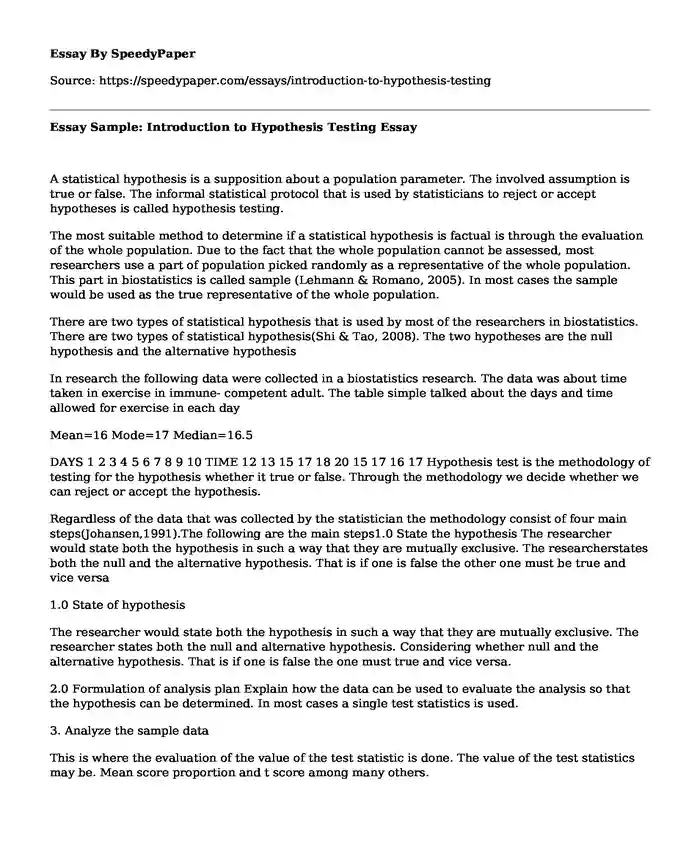# Essay Sample: Introduction to Hypothesis Testing

Published: 2019-05-16Categories: Research Statistics Pages: 3 Wordcount: 645 words
143 views

A statistical hypothesis is a supposition about a population parameter. The involved assumption is true or false. The informal statistical protocol that is used by statisticians to reject or accept hypotheses is called hypothesis testing.

Is your time best spent reading someone else’s essay? Get a 100% original essay FROM A CERTIFIED WRITER!

The most suitable method to determine if a statistical hypothesis is factual is through the evaluation of the whole population. Due to the fact that the whole population cannot be assessed, most researchers use a part of population picked randomly as a representative of the whole population. This part in biostatistics is called sample (Lehmann & Romano, 2005). In most cases the sample would be used as the true representative of the whole population.

There are two types of statistical hypothesis that is used by most of the researchers in biostatistics. There are two types of statistical hypothesis(Shi & Tao, 2008). The two hypotheses are the null hypothesis and the alternative hypothesis

In research the following data were collected in a biostatistics research. The data was about time taken in exercise in immune- competent adult. The table simple talked about the days and time allowed for exercise in each day

Mean=16 Mode=17 Median=16.5

DAYS 1 2 3 4 5 6 7 8 9 10 TIME 12 13 15 17 18 20 15 17 16 17 Hypothesis test is the methodology of testing for the hypothesis whether it true or false. Through the methodology we decide whether we can reject or accept the hypothesis.

Regardless of the data that was collected by the statistician the methodology consist of four main steps(Johansen,1991).The following are the main steps1.0 State the hypothesis The researcher would state both the hypothesis in such a way that they are mutually exclusive. The researcherstates both the null and the alternative hypothesis. That is if one is false the other one must be true and vice versa

1.0 State of hypothesis

The researcher would state both the hypothesis in such a way that they are mutually exclusive. The researcher states both the null and alternative hypothesis. Considering whether null and the alternative hypothesis. That is if one is false the one must true and vice versa.

2.0 Formulation of analysis plan Explain how the data can be used to evaluate the analysis so that the hypothesis can be determined. In most cases a single test statistics is used.

3. Analyze the sample data

This is where the evaluation of the value of the test statistic is done. The value of the test statistics may be. Mean score proportion and t score among many others.

4. Interpret the results

This is where the researcher now decides whether to accept the null hypothesis or reject the null hypothesis by applying the decision rule.

The significant part of any research design and must be tested directly. In definition the null hypothesis may look as the opposite of alternative hypothesis (Wilcox, 2012).The null hypothesis represents the common view of something while the alternative hypothesis is what the researcher dream as the cause of the problem.

In a biostatistics research the researcher may come up with the following hypothesis as null hypothesis

H1:Tomato plant show higher growth rate in loam soil than clay soil

H2: Tomato plant do not show higher growth rate in loam soil than clay soil.

It is important to note that is in statistics the null hypothesis and the alternative hypothesis is very important in the analysis of the statistical data. The null hypothesis and the alternative hypothesis are used with other.

Reference

Shi, N.-Z., & Tao, J. (2008). Statistical hypothesis testing: Theory and methods. Singapore: World Scientific Pub.(Shi & Tao, 2008)

Wilcox, R. R. (2012). Introduction to robust estimation and hypothesis testing. London: Academic.(Wilcox,2012)

Lehmann, E. L., & Romano, J. P. (2005).Testing statistical hypotheses. New York: Springer.(Lehmann & Romano,2005)

Johansen, S. (1991). Estimation and hypothesis testing of cointegration vectors in Gaussian vector autoregressive models. Econometrica: Journal of the Econometric Society, 1551-1580.(Johansen,1991)

Essay Sample: Introduction to Hypothesis Testing. (2019, May 16). Retrieved from https://speedypaper.com/essays/introduction-to-hypothesis-testing

Request Removal

If you are the original author of this essay and no longer wish to have it published on the SpeedyPaper website, please click below to request its removal:

Liked this essay sample but need an original one?

Hire a professional with VAST experience!